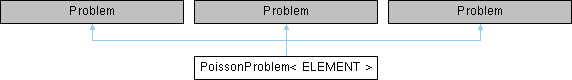PoissonProblem< ELEMENT > Class Template Reference

2D Poisson problem on rectangular domain, discretised with 2D QPoisson elements. The specific type of element is specified via the template parameter. More...

Inheritance diagram for PoissonProblem< ELEMENT >:## Public Member Functions

PoissonProblem (PoissonEquations< 2 >::PoissonSourceFctPt source_fct_pt)
Constructor: Pass pointer to source function. More...

~PoissonProblem ()
Destructor (empty) More...

void actions_before_newton_solve ()
Update the problem specs before solve: Reset boundary conditions to the values from the exact solution. More...

void actions_after_newton_solve ()
Update the problem after solve (empty) More...

void doc_solution (DocInfo &doc_info)
Doc the solution. DocInfo object stores flags/labels for where the output gets written to. More...

PoissonProblem (PoissonEquations< 2 >::PoissonSourceFctPt source_fct_pt)
Constructor: Pass pointer to source function. More...

~PoissonProblem ()
Destructor (empty) More...

void actions_before_newton_solve ()
Update the problem specs before solve: Reset boundary conditions to the values from the exact solution. More...

void actions_after_newton_solve ()
Update the problem after solve (empty) More...

void doc_solution (DocInfo &doc_info)
Doc the solution. DocInfo object stores flags/labels for where the output gets written to. More...

PoissonProblem (PoissonEquations< 2 >::PoissonSourceFctPt source_fct_pt, const unsigned &nel_1d, const bool &mess_up_order)
Constructor: Pass pointer to source function and flag to indicate if order of elements should be messed up. nel_1d is the number of elements along the 1d mesh edges – total number of elements is nel_1d x nel_1d. More...

~PoissonProblem ()
Destructor (empty) More...

void actions_before_newton_solve ()
Update the problem specs before solve: Reset boundary conditions to the values from the exact solution. More...

void actions_after_newton_solve ()
Update the problem after solve (empty) More...

void doc_solution (DocInfo &doc_info)
Doc the solution. DocInfo object stores flags/labels for where the output gets written to. More...

## Private Attributes

PoissonEquations< 2 >::PoissonSourceFctPt Source_fct_pt
Pointer to source function. More...

## Detailed Description

template<class ELEMENT>
class PoissonProblem< ELEMENT >

2D Poisson problem on rectangular domain, discretised with 2D QPoisson elements. The specific type of element is specified via the template parameter.

Definition at line 84 of file two_d_poisson.cc.

## ◆ PoissonProblem() [1/3]

template<class ELEMENT >
 PoissonProblem< ELEMENT >::PoissonProblem ( PoissonEquations< 2 >::PoissonSourceFctPt source_fct_pt )

Constructor: Pass pointer to source function.

Constructor for Poisson problem: Pass pointer to source function.

Definition at line 120 of file two_d_poisson.cc.

References PoissonProblem< ELEMENT >::Source_fct_pt.

## ◆ ~PoissonProblem() [1/3]

template<class ELEMENT >
 PoissonProblem< ELEMENT >::~PoissonProblem ( )
inline

Destructor (empty)

Definition at line 93 of file two_d_poisson.cc.

## ◆ PoissonProblem() [2/3]

template<class ELEMENT >
 PoissonProblem< ELEMENT >::PoissonProblem ( PoissonEquations< 2 >::PoissonSourceFctPt source_fct_pt )

Constructor: Pass pointer to source function.

## ◆ ~PoissonProblem() [2/3]

template<class ELEMENT >
 PoissonProblem< ELEMENT >::~PoissonProblem ( )
inline

Destructor (empty)

Definition at line 95 of file two_d_poisson2.cc.

## ◆ PoissonProblem() [3/3]

template<class ELEMENT >
 PoissonProblem< ELEMENT >::PoissonProblem ( PoissonEquations< 2 >::PoissonSourceFctPt source_fct_pt, const unsigned & nel_1d, const bool & mess_up_order )

Constructor: Pass pointer to source function and flag to indicate if order of elements should be messed up. nel_1d is the number of elements along the 1d mesh edges – total number of elements is nel_1d x nel_1d.

Constructor for Poisson problem: Pass pointer to source function and a flag that specifies if the order of the elements should be messed up. nel_1d is the number of elements along the 1d mesh edges – total number of elements is nel_1d x nel_1d.

Definition at line 127 of file two_d_poisson_compare_solvers.cc.

References PoissonProblem< ELEMENT >::Source_fct_pt.

## ◆ ~PoissonProblem() [3/3]

template<class ELEMENT >
 PoissonProblem< ELEMENT >::~PoissonProblem ( )
inline

Destructor (empty)

Definition at line 97 of file two_d_poisson_compare_solvers.cc.

## ◆ actions_after_newton_solve() [1/3]

template<class ELEMENT >
 void PoissonProblem< ELEMENT >::actions_after_newton_solve ( )
inline

Update the problem after solve (empty)

Definition at line 100 of file two_d_poisson.cc.

## ◆ actions_after_newton_solve() [2/3]

template<class ELEMENT >
 void PoissonProblem< ELEMENT >::actions_after_newton_solve ( )
inline

Update the problem after solve (empty)

Definition at line 102 of file two_d_poisson2.cc.

## ◆ actions_after_newton_solve() [3/3]

template<class ELEMENT >
 void PoissonProblem< ELEMENT >::actions_after_newton_solve ( )
inline

Update the problem after solve (empty)

Definition at line 104 of file two_d_poisson_compare_solvers.cc.

## ◆ actions_before_newton_solve() [1/3]

template<class ELEMENT >
 void PoissonProblem< ELEMENT >::actions_before_newton_solve

Update the problem specs before solve: Reset boundary conditions to the values from the exact solution.

Update the problem specs before solve: (Re-)set boundary conditions to the values from the exact solution.

Definition at line 183 of file two_d_poisson.cc.

References TanhSolnForPoisson::get_exact_u().

## ◆ actions_before_newton_solve() [2/3]

template<class ELEMENT >
 void PoissonProblem< ELEMENT >::actions_before_newton_solve ( )

Update the problem specs before solve: Reset boundary conditions to the values from the exact solution.

## ◆ actions_before_newton_solve() [3/3]

template<class ELEMENT >
 void PoissonProblem< ELEMENT >::actions_before_newton_solve ( )

Update the problem specs before solve: Reset boundary conditions to the values from the exact solution.

## ◆ doc_solution() [1/3]

template<class ELEMENT >
 void PoissonProblem< ELEMENT >::doc_solution ( DocInfo & doc_info )

Doc the solution. DocInfo object stores flags/labels for where the output gets written to.

Doc the solution: doc_info contains labels/output directory etc.

Definition at line 221 of file two_d_poisson.cc.

References TanhSolnForPoisson::get_exact_u().

Referenced by main(), and run().

## ◆ doc_solution() [2/3]

template<class ELEMENT >
 void PoissonProblem< ELEMENT >::doc_solution ( DocInfo & doc_info )

Doc the solution. DocInfo object stores flags/labels for where the output gets written to.

## ◆ doc_solution() [3/3]

template<class ELEMENT >
 void PoissonProblem< ELEMENT >::doc_solution ( DocInfo & doc_info )

Doc the solution. DocInfo object stores flags/labels for where the output gets written to.

## ◆ Source_fct_pt

template<class ELEMENT >
 PoissonEquations< 2 >::PoissonSourceFctPt PoissonProblem< ELEMENT >::Source_fct_pt
private

Pointer to source function.

Definition at line 109 of file two_d_poisson.cc.

Referenced by PoissonProblem< ELEMENT >::PoissonProblem().

The documentation for this class was generated from the following files: